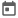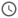# Clocking blocks in SV##### 22 Jul 2023##### 6 mins

Clocking blocks are a special block introduced in System Verilog which can be used to get synchronized view of set of signals with regards to a clock. This also helps to makes it easier to access the signal from other components like monitor and driver. Let’s explore clocking block in detail in this article.

### Introduction

#### What are clocking blocks?

Clocking block as name suggests are a block which are used to synchronise signal with a clock. We know that in sequential circuits clocks play an important role and all operation happens at some edge of the clock. Thus, driving the signal and sampling it is crucial from the test bench to avoid race conditions. If there are issues in how we drive and sample signals, we can miss some bugs in rtl.

#### Purpose of Clocking Blocks

Let’s see why clocking block is needed.

• Signal Synchronization – clocking blocks helps provide an environment whose components are synchronized. Wherever we use a signal from a clocking block, it is guaranteed that the signal would be driven or sampled at the same clock edge.
• Time aware verification – clocking blocks helps in time-aware verification which is crucial mainly when we are verifying interface. As signals are synchronized in clocking block, these signals can be used to write assertions, function coverage which would be prone to any race condition samplings.
• Prevent metastability issues – Metastability refers to the situation where a signal does not have a well-defined value. This generally happens near clock edge where due to transitioning and in cross-domain clock scenarios. Clocking block provides a well-structured environment where we can control at point we want to sample/drive a signal thus eliminating metastability issues.

Above points might seems overwhelming but clocking block is something which would be needed frequently in verification. Now let’s move towards the basic building block of clocking blocks.

### Structure of clocking block

#### Clocking block declaration

Clocking blocks are generally declared inside an interface and can be accessed from other components of test bench through interface.

Clocking block is declared using `clocking` keyword and ended using `endclocking`. We also pass the clock with which we want all the containing signals to be synchronized.

``````clocking abc @(posedge clk)
<signal goes here>
endclocking``````

#### Clock Skew

Sequential circuit works on some clock edge. In simulation, during a clock edge the sampled value is the last value of the signal. Thus, the design will behave according to this sampled value. Lets suppose we are driving some signal in the posedge of the clock and the design is also sensitive to the positive edge of the clock, thus design will not respond to the new value, rather it will take previous value. This can create confusion in verification, as the expected output from the design should be according to new value.

Clocking block provides a way by which we can provide skew to the signals. That is, it will be sampled few time unit before clock edge and driven few time units after clock edge. This ensures that test bench and design are in sync and correct output is validated for correct inputs.

There are 2 ways to provide clock skew in clocking blocks.

• Default clock skew – `default` keyword is used to define a default clock skew for all the signals inside the clocking block, despite it is input or output.
• Individual clock skew – we can even provide individual clock skew to the signals or override default values for some signals. Individual clock skew are provided when we define the direction of the signal using `input`, `output` keywords.

We will see how to provide clock skews in example below.

``````clocking abc @(posedge clk);
default input #1 output #1; //gives a default skew of 1 timeunit to all signals
input a, b;
output #2 c; // overrides the skew of output c to 2 timeunits
endclocking``````
The thing which we must remember here is that skew for output signal means the timeunits after clock edge in which signal will be driven whereas for input signals it means the timeunits before clock edge in which signal will be sampled.

In this section we will discuss the advantage of clocking block.

• Helps in handling clock skew and jitter – clock skew can happen a edge of the clock arrives at different area of chip at different time. As clocking block provides a synchronized environment for all signals in same clock domain, thus skew is avoided. Jitter refers to minor variations in the clock period. In real chip it is hard to get a perfect clock and all clocks will have minor variations in the clock period. As clocking blocks sample clock in the stable clock, it reduces risk of capturing wrong value.
• Time aware verification – We have already discussed how clocking blocks help in time aware verification by providing a synchronized environment.
• Testbench Development Simplification – Clocking block simplifies the test bench as once we have declared the clocking block, we can use this in any of the components without worrying about the clock.
• Prevents race condition – Clocking block provides an in-built event which triggers every time a clock edge arrives. If inside test bench we could directly use the clock edge in sequential block it can cause race condition. By using the event provided by clocking block in seq. block we ensure that the block is triggered without any race condition.

Enough of the theories, now let’s see some practical scenarios.

### Usage Examples

In this section we will see some practical examples of clocking block.

#### Basic scenario with skews

In any test bench, we would have a driver which will drive input signals and a monitor which will sample all the signals. In this example we will declare 2 clocking blocks, one for monitor and one for driver and provide different skews to the signals. This will help realize how skew actually works and their practical significance.

``````module dut(input clk, a, b, output reg c);
always @(posedge clk)
c <= a + b;
endmodule

module tb;
bit clk;
reg a, b;
wire c;

always #10 clk = ~clk;

dut d1(.clk(clk), .a(a), .b(b), .c(c));

clocking drv_cb @(posedge clk);
default input #1 output #1;
output a, b;
endclocking

clocking mon_cb_1 @(posedge clk);
default input #1step;
input a, b ,c;
endclocking

clocking mon_cb_2 @(posedge clk);
default input #1;
input a, b;
input #0 c;
endclocking

initial begin
drv_cb.a <= 1;
drv_cb.b <= 0;
#35;
drv_cb.a <= 0;
drv_cb.b <= 1;
end

always @(mon_cb_1) begin
\$display("[%0t] sampled from mon cb_1: a = %0b, b = %0b, c = %0b",\$time, mon_cb_1.a, mon_cb_1.b, mon_cb_1.c);
\$display("[%0t] sampled from mon cb_2: a = %0b, b = %0b, c = %0b",\$time, mon_cb_2.a, mon_cb_2.b, mon_cb_2.c);
end
endmodule``````
##### Output
``````#  sampled from mon cb_1: a = x, b = x, c = x
#  sampled from mon cb_2: a = x, b = x, c = x
#  sampled from mon cb_1: a = 1, b = 0, c = x
#  sampled from mon cb_2: a = 1, b = 0, c = 1
#  sampled from mon cb_1: a = 1, b = 0, c = 1
#  sampled from mon cb_2: a = 1, b = 0, c = 1
#  sampled from mon cb_1: a = 0, b = 1, c = 1
#  sampled from mon cb_2: a = 0, b = 1, c = 1
#  sampled from mon cb_1: a = 0, b = 1, c = 1
#  sampled from mon cb_2: a = 0, b = 1, c = 1``````
##### WaveWaveform for the above example. [Courtesy - Eda Playground]Try this code in EDA Playground

In above waveform we can see that the signals are driven 1ns after the clock edge as we have provided skew of 1timeunit. Also, when we are driving the signal after 35ns, it is driven in the next clock cycle near 50ns. Thus, clocking block synchronizes the signal to a clock.

In the output, we can see that the mon_cb_2 prints the actual output from the design whereas mon_cb_1 prints the previous output from design. This is because we use #0 as skew in the mon_cb_2 and thus, sampling happens in the non-active region. This is how we can use different skews to sample signals at different time.

#### Cross domain clock verification

Cross domain clock (cdc) designs are common nowadays as complex circuit can work on more than 1 clock to increase efficiency.

In this example, we will verify a simple design which works on 2 clocks, clk1 for input and clk2 for output. Input of the design is `clk1`, `clk2`, `rst`, `a`, `b`, `req` and output are `c` and `busy`. When dut receives `req`, it will output the sum of `a` and `b` in `c`. `busy` will be high until `c` is latched with the correct value as output are working on clk2.

``````module cdc_dut(input clk1, clk2, rst, a, b, req, output reg c, busy);
reg temp_sum;

always @(negedge rst) begin
if(~rst) begin
temp_sum <= 0;
busy <= 0;
end
end
always @(posedge clk1) begin
if(~busy && req) begin
temp_sum <= a + b;
busy <= 1;
end
end

always @(posedge clk2) begin
c = temp_sum;
busy <= 0;
end
endmodule

module tb;
bit clk1, clk2;
reg a, b, rst, req;
wire c, busy;
always #10 clk1 = ~clk1;
always #25 clk2 = ~clk2;

cdc_dut dut(clk1, clk2, rst, a, b, req, c, busy);

clocking drv_cb @(posedge clk1);
default input #1step output #1;
output a, b, req;
input busy;
endclocking

clocking mon_cb_1 @(posedge clk1);
input #1 a, b, req, busy;
endclocking

clocking mon_cb_2 @(posedge clk2);
input #0 c, busy;
endclocking

// Driver
initial begin
//reset packet
rst <= 0;
drv_cb.req <= 0;
drv_cb.a <= 0;
drv_cb.b <= 0;
@(drv_cb);
wait(~mon_cb_2.busy);
#10;
rst <= 1;
// 1st valid ip
drv_cb.req <= 1;
drv_cb.a <= 0;
drv_cb.b <= 1;
@(drv_cb);
wait(~mon_cb_2.busy);
@(drv_cb);
drv_cb.req <= 0;
@(drv_cb);
#40;
//2nd valid ip
drv_cb.req <= 1;
drv_cb.a <= 1;
drv_cb.b <= 1;
@(drv_cb);
wait(~mon_cb_2.busy);
@(drv_cb);
drv_cb.req <= 0;
@(drv_cb);
#40;
//3rd valid ip
drv_cb.req <= 1;
drv_cb.a <= 1;
drv_cb.b <= 0;
@(drv_cb);
wait(~mon_cb_2.busy);
@(drv_cb);
drv_cb.req <= 0;
end

// Monitor (checker)
always @(posedge mon_cb_1.req) begin
forever begin
@(mon_cb_2);
if(~mon_cb_2.busy) break;
end
if(~mon_cb_2.busy) begin
if(mon_cb_1.a + mon_cb_1.b == mon_cb_2.c )
\$display("[%0t] Output is expected. a = %0b, b = %0b, c = %0b", \$time, mon_cb_1.a, mon_cb_1.b, mon_cb_2.c);
else
\$display("[%0t] Output is not expected. a = %0b, b = %0b, c = %0b", \$time, mon_cb_1.a, mon_cb_1.b, mon_cb_2.c);
end
end
endmodule``````
##### Output
``````#  Output is expected. a = 0, b = 1, c = 1
#  Output is expected. a = 1, b = 1, c = 0
#  Output is expected. a = 1, b = 0, c = 1``````
Try this code in EDA Playground
In all our example, we used clocking blocks directly inside a module. But best practice is to define inside an interface as most of out test bench components will be class and not module. Also, defining clocking block inside interface helps to encapsulate better.

### Conclusion

Clocking block is an important concept in System Verilog and very useful for verification as we have seen in this article. There are numerous use cases of clocking block which is hard to cover in a single article. Readers are suggested to explore more on the use cases by developing different test benches.